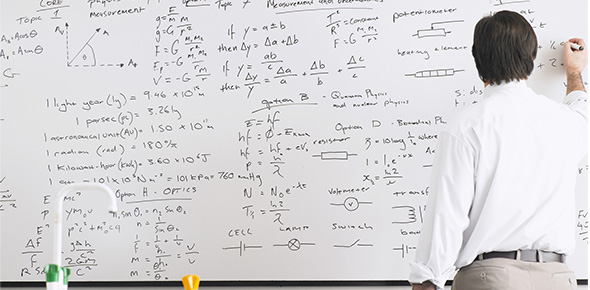# Problem Solving Skills

12 Questions | Total Attempts: 72SettingsAre you any good at problem-solving, and think you can pass this quiz? Problem-solving necessitates a systematic step by step approach to a challenge. Problem-solving is considered a personal strength rather than a skill that is learned through education and instruction. You can improve your problem-solving skills by learning from a more experienced teacher. If you want to become better with the problem-solving skills, then try this quiz.

• 1.
Mrs. Straley bought 2 hats and a microwave. One hat cost \$5.98, and the other hat cost \$8.97. The microwave cost \$97.88. What was the difference between the cost of the 2 hats and the cost of the sofa?
• A.

\$82.93

• B.

\$91.90

• C.

\$14.95

• D.

\$89.01

• 2.
At a grocery store, the were 180 cereal boxes on shelves. There were 6 different brands of cereal, and there were an equal number of boxes of each brand. What is a way of determining the number of cereal boxes of each brand on the shelves?
• A.

Subtracct 6 from 180

• B.

• C.

Multiply 180 by 6

• D.

Divide 180 by 6

• 3.
Olga bought 25 T-shirts for \$8 each, including tax. She sold them all for \$12 each. What is the difference between the amount of money Olga made and the amount of money she spent on these 25 T-shirts?
• A.

\$500

• B.

\$100

• C.

\$20

• D.

\$200

• 4.
Mrs. Kline was putting wallpaper on her kitchen walls. She used 5 rolls of wallpaper and 2 feet of another roll to cover half the kitchen. What information is needed to find the total number of feet of wallpaper Mrs. Kline needed to cover the whole kitchen?
• A.

The number of rolls of wallpaper she bought

• B.

The cost of a roll of wallpaper

• C.

The number of feet on each roll of wallpaper

• D.

The number of feet of wallpaper left over when she finishes the kitchen

• 5.
Sarah and Jen participated in the Frisbee toss on field day. Sarah threw the Frisbee 30.95 meters. Jen threw the Frisbee 39.31 meters. How much farther did Jen throw the Frisbee than Sarah?
• A.

9.64 m

• B.

8.36 m

• C.

8.64 m

• D.

9.36 m

• 6.
A group of students made a total of \$672 by washing cars on Saturday. They charged \$16 to wash each car. How many cars did they wash?
• A.

656

• B.

40

• C.

688

• D.

42

• 7.
A high school principal ordered 244 boxes of black ink pens, 497 boxes of red ink pens, and 352 boxes of pencils for her teachers. Which of these shows the number of boxes of ink pens the principal ordered?
• A.

The difference between 497 and 244

• B.

The sum of 497, 352, and 244

• C.

The sum of 497 and 244

• D.

The difference between 497 and 352

• 8.
Cedric, denzel, and Tyson bought a pacckage of 36 pens for \$6. Which of these shows the number of pens each boy will get if they share the pens equally?
• A.

The product of 36 and 3

• B.

The product of 36 and 6

• C.

The quotient of 36 and 6

• D.

The quotient of 36 and 3

• 9.
A toy store received 60 dolls and 36 teddy bears on Monday. The dolls arrived in boxes with 12 dolls in each box. The teddy bears ccame with 4 in each box. Which of the following can be used to find how many boxes of dolls the store received?
• A.

Divide 60 by 12

• B.

Multiply 60 by 12

• C.

Divide 60 by 4

• D.

Multiply 36 by 12

• 10.
Cordell received \$60 as a gift. He bought 5 books that cost \$10 each. Which of the following shows a way to find the amount of money Cordell had then?
• A.

Subtract 10 from 60 and then multiply the difference by 5

• B.

Multiply 5 by 10 and then subtract the product from 60

• C.

Subtract 10 from 60 and then divide the difference by 5

• D.

Divide 10 by 5 and then subtract the quotient from 60

• 11.
A worker had 159 green tiles and 434 blue tiles to be used to tile the floor of a restaurant. When he finished the floor, there were 97 blue tiles that he did not use. Which of the following should the worker do to find how many tiles he used on the restaurant floor?
• A.

Subtract 159 from the sum of 434 and 97

• B.

Subtract 97 fromt he sum of 159 and 434

• C.

Subtract 434 from the sum of 159 and 97

• D.

Add 97 to the difference between 434 and 159

• 12.
Julieta sold her dollhouse for \$75. She worked 25 hours at her father's store last week andwas paid \$5 per hour. Which of the following should Julieta do first to find how much money she has now?
• A.

Multiply 25 by 5

• B.